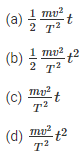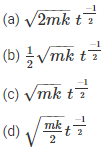# Work, Energy and Power MCQ for NEET

NEET  Physics is the scoring paper in the medical entrance examination. Here, you will discover the NEET Physics MCQ Questions for all Concepts as per the latest syllabus. Practice more on a regular basis with these NEET Physics objective questions on air pollution and improve your subject knowledge & problem-solving skills along with time management. NEET Physics Work, Energy and Power Multiple Choice Questions make you feel confident in answering the question in the exam & increases your scores to high.

## MCQ on Work, Energy and Power

1. By how much does kinetic energy increase if the momentum is increased by 20%
(a) 55%
(b) 66%
(c) 44%
(d) 77%

2. Calculate the energy loss in a perfectly inelastic collision if the mass of the object is 40kg with velocity 4m/s hits the object of mass 60kg with velocity 2m/s.
(a) 440J
(b) 110J
(c) 392J
(d) 48J

3. Calculate the final kinetic energy of a block of mass if the mass of the block is 10kg and has a constant velocity of 10m/s. The block of mass is subjected to a retarding force of F=0.1J/m
(a) 275J
(b) 250J
(c) 475J
(d) 450J

4. What is the average power required to lift a mass of 100kg to a height of 50m in 50 seconds?
(a) 980
(b) 100
(c) 50
(d) 5000

5. What is the power of the engine when the velocity of the car is v, mass m, acceleration a, and external resistance R
(a) (R-ma)v
(b) (R+ma)v
(c) mav
(d) Rv

6. Consider an object with m as its mass such that it is accelerated uniformly from rest and the speed attained by the object is v in T time.  Calculate the instantaneous power that is delivered to the body in terms of function of timeAnswer: (b) $$\frac{1}{2} \frac{m v^{2}}{T^{2}} t^{2}$$

7. A ball is thrown from a height of 20 m vertically downward and has an initial velocity of $$v_{0}^{2}$$. As the stone collides with the ground 50% of its energy is lost and bounce back to the same height. What is the initial velocity?
(a) 28m/s
(b) 20m/s
(c) 10m/s
(d) 14m/s

8. Consider a particle of mass m which is driven by a machine that has a constant power k Watts. What is the force on the particle at time t if the particle starts from the rest?Answer: (d) $$\sqrt{\frac{m k}{2}} t^{\frac{-1}{2}}$$

9. When can one say that work is done on the body
(a) When the body experiences force
(b) When there is an increase in energy because of mechanical influence
(c) When the body moves a certain distance
(d) None of the above

Answer: (b) When there is an increase in energy because of mechanical influence

10. Select the incorrect statement for the given situation: Two bodies with mass m1 and m2 undergo elastic collision
(a) When m1 and m2 is at rest, the kinetic energy transferred will be maximum
(b) The bodies will move in the same direction if m1 = m2 and when the collision is in oblique
(c) When m> m2, m2 will move with four times increase in its velocity than m1
(d) When m1 < m2, the transfer of momentum will be maximum

Answer: (c) When m1 > m2, m2 will move with four times increase in its velocity than m1

11. What is the ratio of velocities of a nucleus that splits into two parts with radii 1:2
(a) 6:1
(b) 2:1
(c) 8:1
(d) 4:1Answer: (d) $$P=\frac{d E}{d V}$$